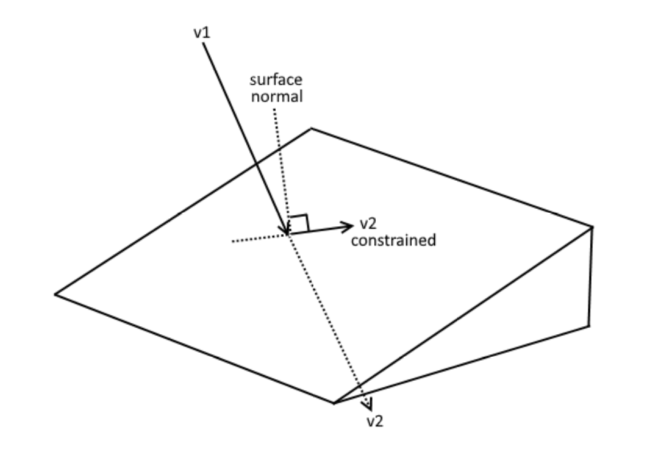# Constraining a vector3 to a surface/plane

This is just a math problem I’m not sure how to do correctly, I have a thing moving through the air and when it runs into a surface (detected with a raycast) I’d like it to stick to the surface and keep moving in the same general direction. The more extreme the hit angle, the more speed is lost.Best I could do was a weird solution that takes a CFrame on the surface and compares the angle difference between its lookVector and the incoming objects velocity then tries to rotate the velocity by the difference but that leaves the velocity at its original magnitude and the angle still isn’t perfect

1 Like

I think all you have to do is subtract off any velocity which is in the direction of the surface normal. If `velocity` is your velocity, and `normal` is the surface normal, it would be:

``````local constrainedVelocity = velocity + normal * velocity:Dot(normal);
``````
4 Likes

I can’t say for sure if that works because I broke some other things, but based on me googling what a dot product is, that should work nicelyWhen I do anything with geometry, I’m “printing” my vectors all the time.

``````--let point be the intersection point
Draw{Ray.new(point, normal)};
Draw{Ray.new(point, velocity)};
Draw{Ray.new(point, constrainedVelocity)};
Draw{Ray.new(point, -normal * velocity:Dot(normal))};
``````

It’s definitely possible my magnitudes are off, and it’s easiest to diagnose a problem if you see what all the intermediate steps are.